# AlgebraPage 4

#### WATCH ALL SLIDES

Answer: Substitution Property of Equality

Slide 44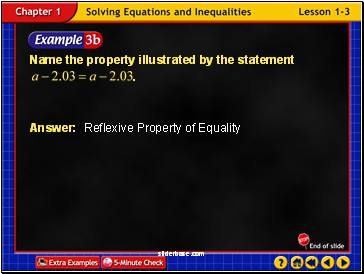Example 3-3b

Answer: Reflexive Property of Equality

Slide 45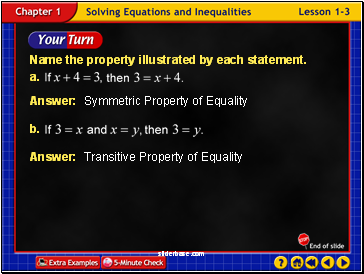Answer: Transitive Property of Equality

Example 3-3c

Answer: Symmetric Property of Equality

Slide 46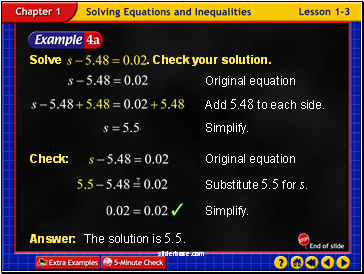Example 3-4a

Answer: The solution is 5.5.

Slide 47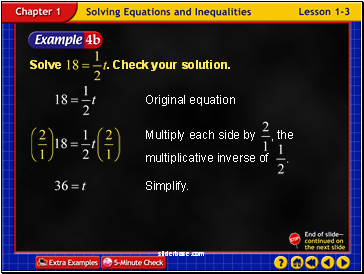Example 3-4b

Slide 48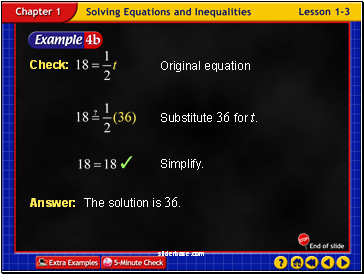Example 3-4c

Answer: The solution is 36.

Slide 49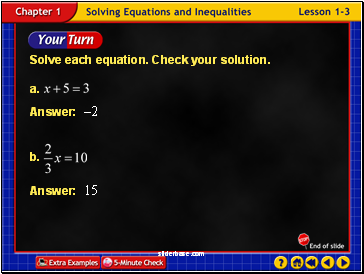Example 3-4d

Slide 50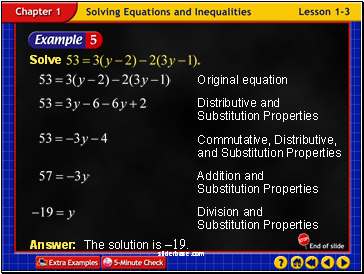Example 3-5a

Answer: The solution is –19.

Slide 51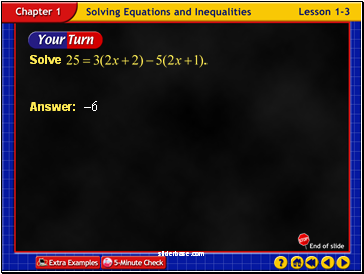Example 3-5b

Slide 52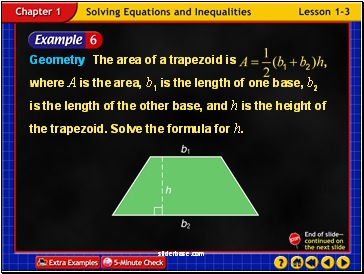Example 3-6a

Slide 53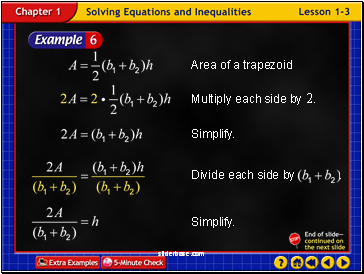Example 3-6b

Slide 54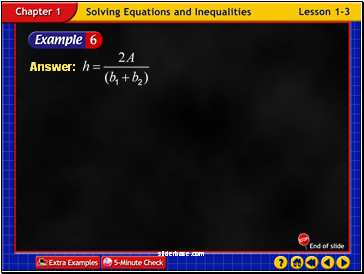Example 3-6c

Slide 55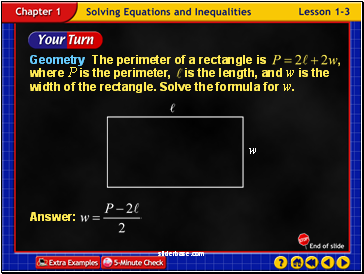Example 3-6d

Slide 56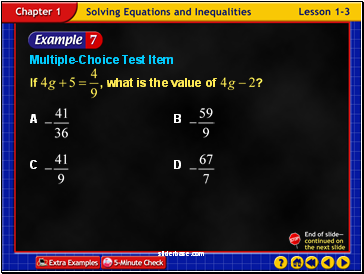Example 3-7a

Slide 57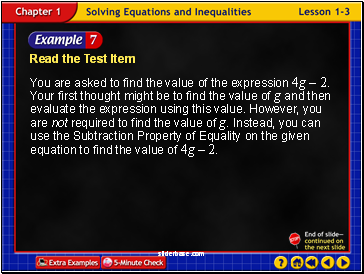Example 3-7b

Read the Test Item

You are asked to find the value of the expression 4g – 2. Your first thought might be to find the value of g and then evaluate the expression using this value. However, you are not required to find the value of g. Instead, you can use the Subtraction Property of Equality on the given equation to find the value of 4g – 2.

Slide 58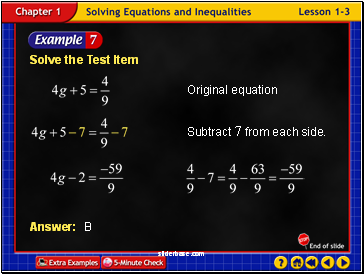Example 3-7c

Solve the Test Item

Slide 59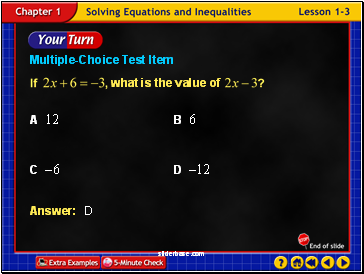Example 3-7d

Slide 60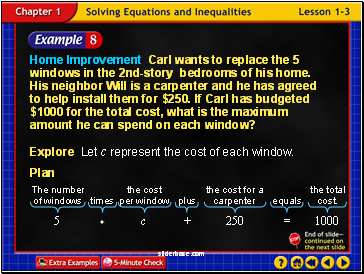Example 3-8a

Home Improvement Carl wants to replace the 5 windows in the 2nd-story bedrooms of his home. His neighbor Will is a carpenter and he has agreed to help install them for \$250. If Carl has budgeted \$1000 for the total cost, what is the maximum amount he can spend on each window?

Go to page:
1  2  3  4  5  6  7  8

## Last added presentations

© 2010-2019 powerpoint presentations